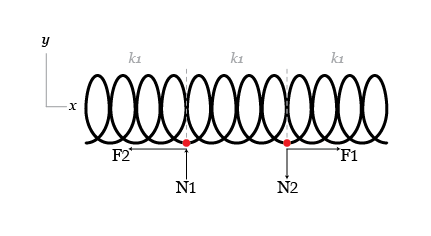# Why we rip the way we do

When tearing a sheet of paper most people exert a force perpendicular to the sheet, rather than grabbing the sheet by the edges and trying to pull it apart. The physics of this can be understood with a simple model.

Consider a row of three springs along the x-axis with the same spring constant and length, connected end to end. Initially, they are all at their natural length. If any of them stretch by more than one percent they will break. This is our paper.Tearing apart is equivalent to putting forces N1 and N2 (each with magnitude N) at the two points where two springs join, in opposite directions and perpendicular to the springs. Pulling apart is equivalent to putting equal and opposite forces F1 and F2 (magnitude F) at the same points but now parallel to the springs. Find the ratio $F/N$ when the middle spring breaks.

Details and assumptions

• Hint 1: Do NOT assume that the ends of the springs move only vertically.
• Hint 2: tear the paper slowly and think about force balances.
×